Function Repository Resource:

# CurveTube

Convert a 3D curve into a parametrized tube

Contributed by: Wolfram Staff (original content by Alfred Gray)
 ResourceFunction["CurveTube"][c,t,r,θ] gives the parametrized circular tube with radius r and cross sectional angle θ centered on the curve c with parameter t. ResourceFunction["CurveTube"][c1,c2,t,r,θ] gives the parametrized tube whose cross section is similar to c2 with effective radius r and cross sectional angle θ centered on the curve c1 with parameter t.

## Details and Options

The parametrization has the form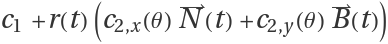, where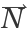and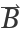are the normal and the binormal vectors of c1, respectively.
The first curve must be 3D and the second must be 2D.
ResourceFunction["CurveTube"] has the same options as ParametricPlot3D.

## Examples

### Basic Examples (3)

Compute the parametrization for a helical tube:

 In:=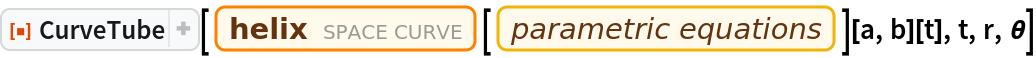Out=Plot the tube:

 In:=Out=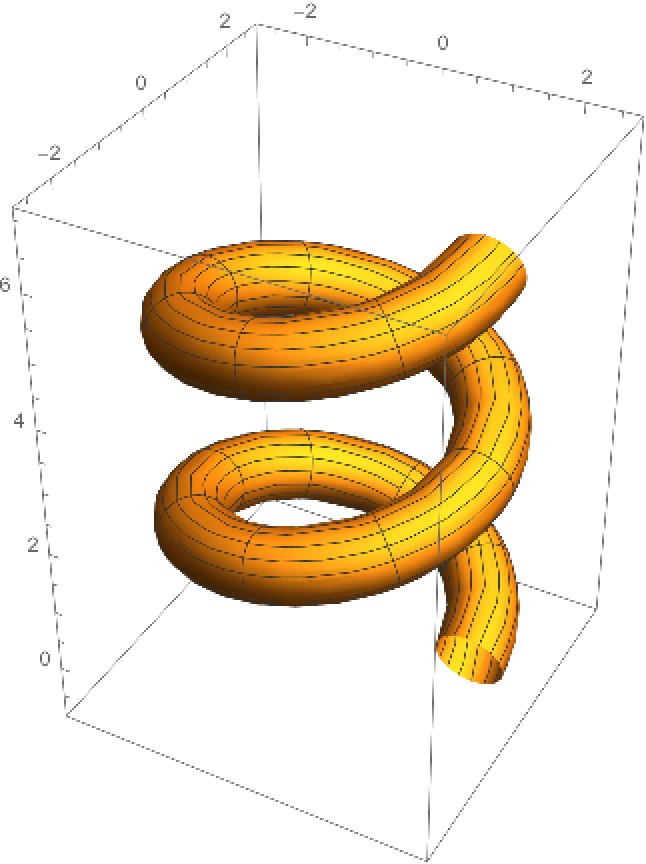Make a tube from a torus knot curve:

 In:=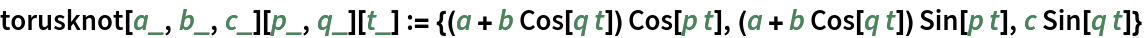In:=Out=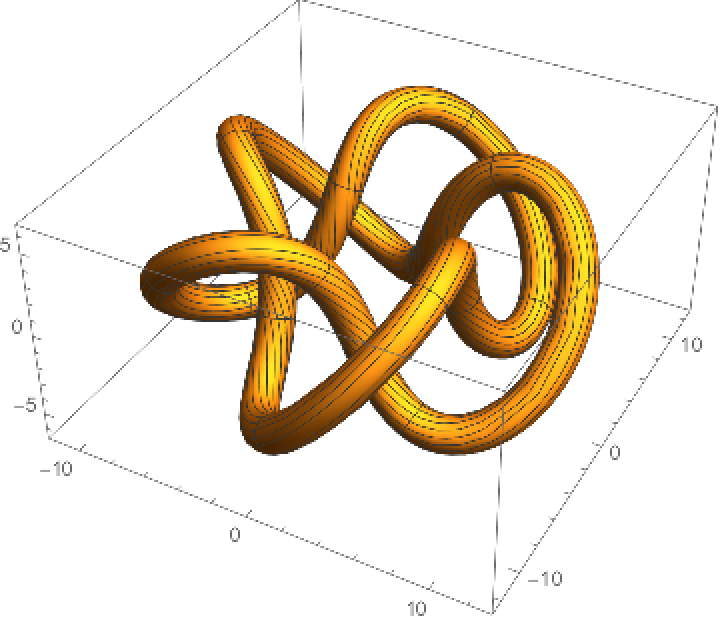Make a helix tube using a variant of a nephroid as a cross section:

 In:=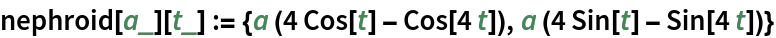In:=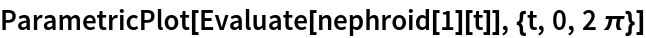Out=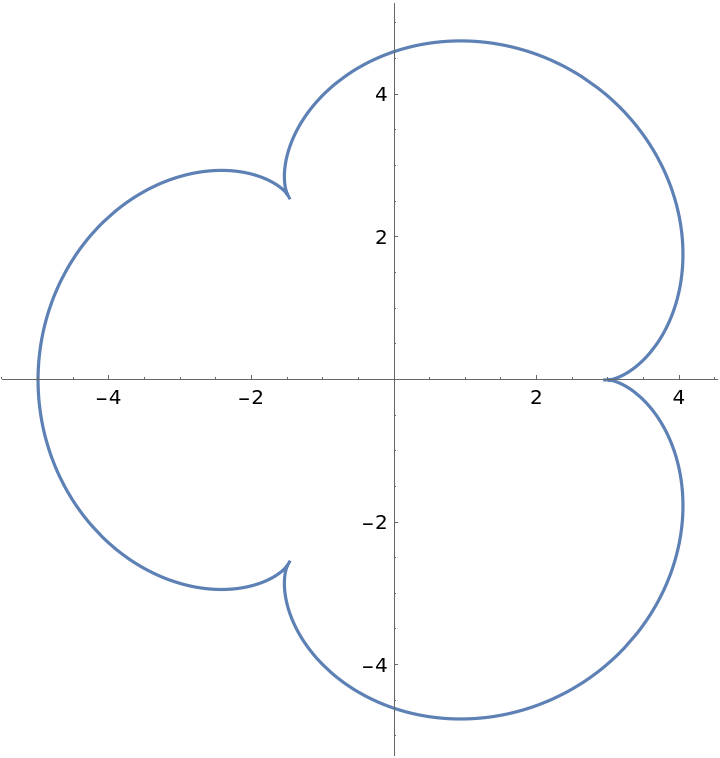In:=Out=In:=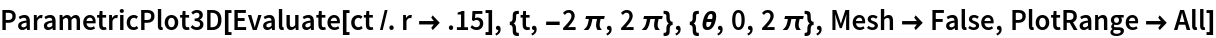Out=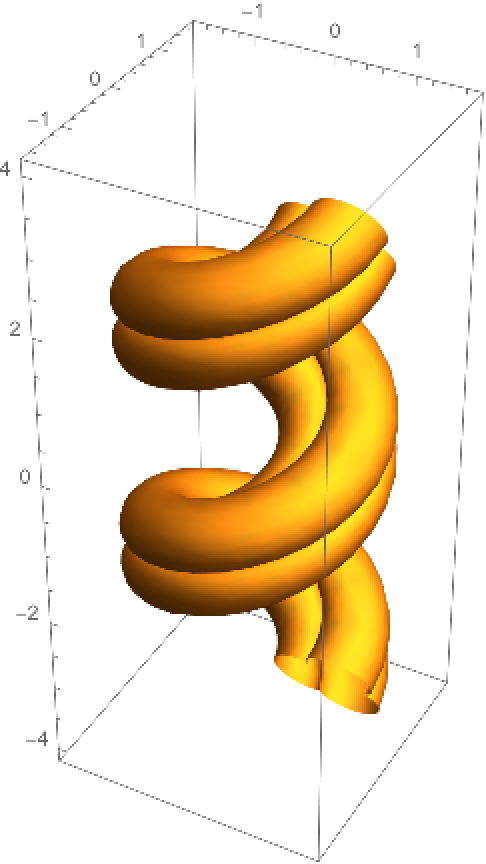### Properties and Relations (2)

Take the normal and binormal vectors from the Frenet-Serret system for a helix:

 In:=Out=The parametrization for the curve tube for a helix is:

 In:=Out=This is the same as given by CurveTube:

 In:=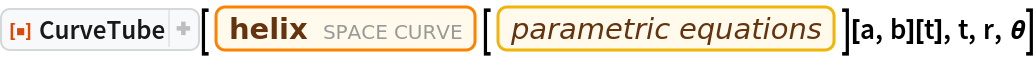Out=Something similar can be done with Tube:

 In:=Out=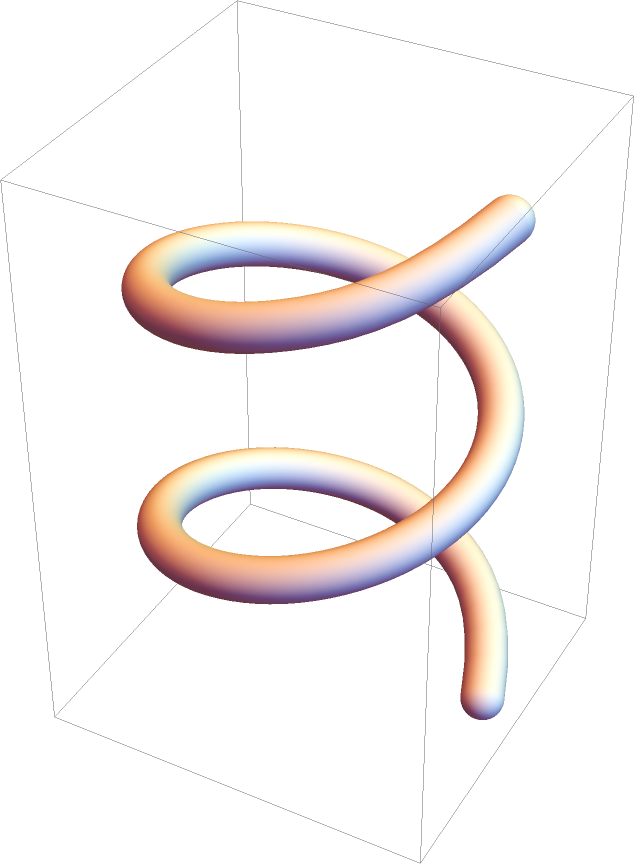Enrique Zeleny

## Version History

• 1.0.0 – 21 July 2020